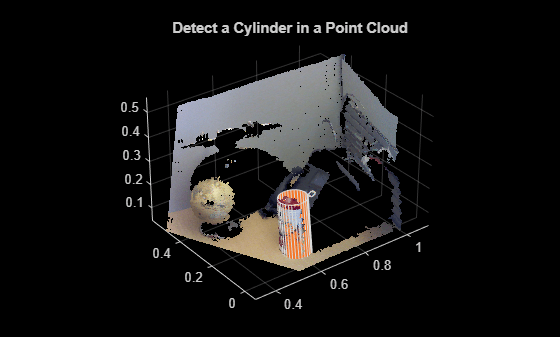# plot

Class: cylinderModel

Plot cylinder in a figure window

## Syntax

``plot(model)``
``plot(model,'Parent',ax)``

## Description

````H` = `plot(model)` plots a cylinder within the axis limits of the current figure. `H` is the handle to `surf`, a 3-D shaded surface plot.```
````H` = `plot(model,'Parent',ax)` additionally specifies an output axes.```

## Input Arguments

expand all

Parametric cylinder model returned by `cylinderModel`.

Output axes, specified as the current axes for displaying the cylinder.

## Examples

expand all

`load('object3d.mat');`

Display point cloud.

```figure pcshow(ptCloud) xlabel('X(m)') ylabel('Y(m)') zlabel('Z(m)') title('Detect a Cylinder in a Point Cloud')```Set the maximum point-to-cylinder distance (5 mm) for the cylinder fitting.

`maxDistance = 0.005;`

Set the region of interest to constrain the search.

```roi = [0.4,0.6;-inf,0.2;0.1,inf]; sampleIndices = findPointsInROI(ptCloud,roi);```

Set the orientation constraint.

`referenceVector = [0,0,1];`

Detect the cylinder in the point cloud and extract it.

```model = pcfitcylinder(ptCloud,maxDistance,referenceVector,... 'SampleIndices',sampleIndices);```

Plot the cylinder.

```hold on plot(model)```